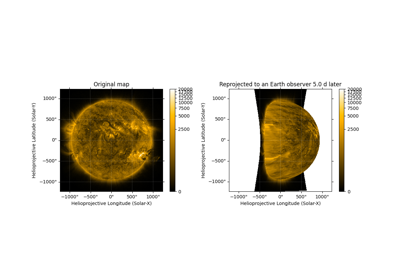# propagate_with_solar_surface¶

sunpy.coordinates.propagate_with_solar_surface(rotation_model='howard')[source]

Context manager for coordinate transformations to automatically apply solar differential rotation for any change in observation time.

Normally, coordinates refer to a point in inertial space (relative to the barycenter of the solar system). Transforming to a different observation time does not move the point at all, but rather only updates the coordinate representation as needed for the origin and axis orientations at the new observation time.

Under this context manager, transformations will instead treat the coordinate as if it were referring to a point on the solar surface instead of a point in inertial space. If a transformation has a change in observation time, the heliographic longitude of the point will be updated according to the specified rotation model.

Parameters:

rotation_model (`str`) – Accepted model names are `'howard'` (default), `'snodgrass'`, `'allen'`, and `'rigid'`. See the documentation for `diff_rot()` for the differences between these models.

Notes

This context manager also ignores the motion of the center of the Sun (see `transform_with_sun_center()`).

Due to the implementation approach, this context manager modifies transformations between only these five coordinate frames: `HeliographicStonyhurst`, `HeliographicCarrington`, `HeliocentricInertial`, `Heliocentric`, and `Helioprojective`.

ExamplesDifferentially rotating a map

Differentially rotating a map
```>>> import astropy.units as u
>>> from astropy.coordinates import SkyCoord
>>> from sunpy.coordinates import HeliocentricInertial, propagate_with_solar_surface
>>> meridian = SkyCoord(0*u.deg, [-60, -30, 0, 30, 60]*u.deg, 1*u.AU,
...                     frame=HeliocentricInertial, obstime='2021-09-15')
>>> out_frame = HeliocentricInertial(obstime='2021-09-21')
>>> with propagate_with_solar_surface():
...     print(meridian.transform_to(out_frame))
<SkyCoord (HeliocentricInertial: obstime=2021-09-21T00:00:00.000): (lon, lat, distance) in (deg, deg, AU)
[(70.24182965, -60., 1.),
(82.09298036, -30., 1.),
(85.9579703 ,   0., 1.),
(82.09298036,  30., 1.),
(70.24182965,  60., 1.)]>
>>> with propagate_with_solar_surface(rotation_model='rigid'):
...     print(meridian.transform_to(out_frame))
<SkyCoord (HeliocentricInertial: obstime=2021-09-21T00:00:00.000): (lon, lat, distance) in (deg, deg, AU)
[(85.1064, -60., 1.), (85.1064, -30., 1.),
(85.1064,   0., 1.), (85.1064,  30., 1.),
(85.1064,  60., 1.)]>
```